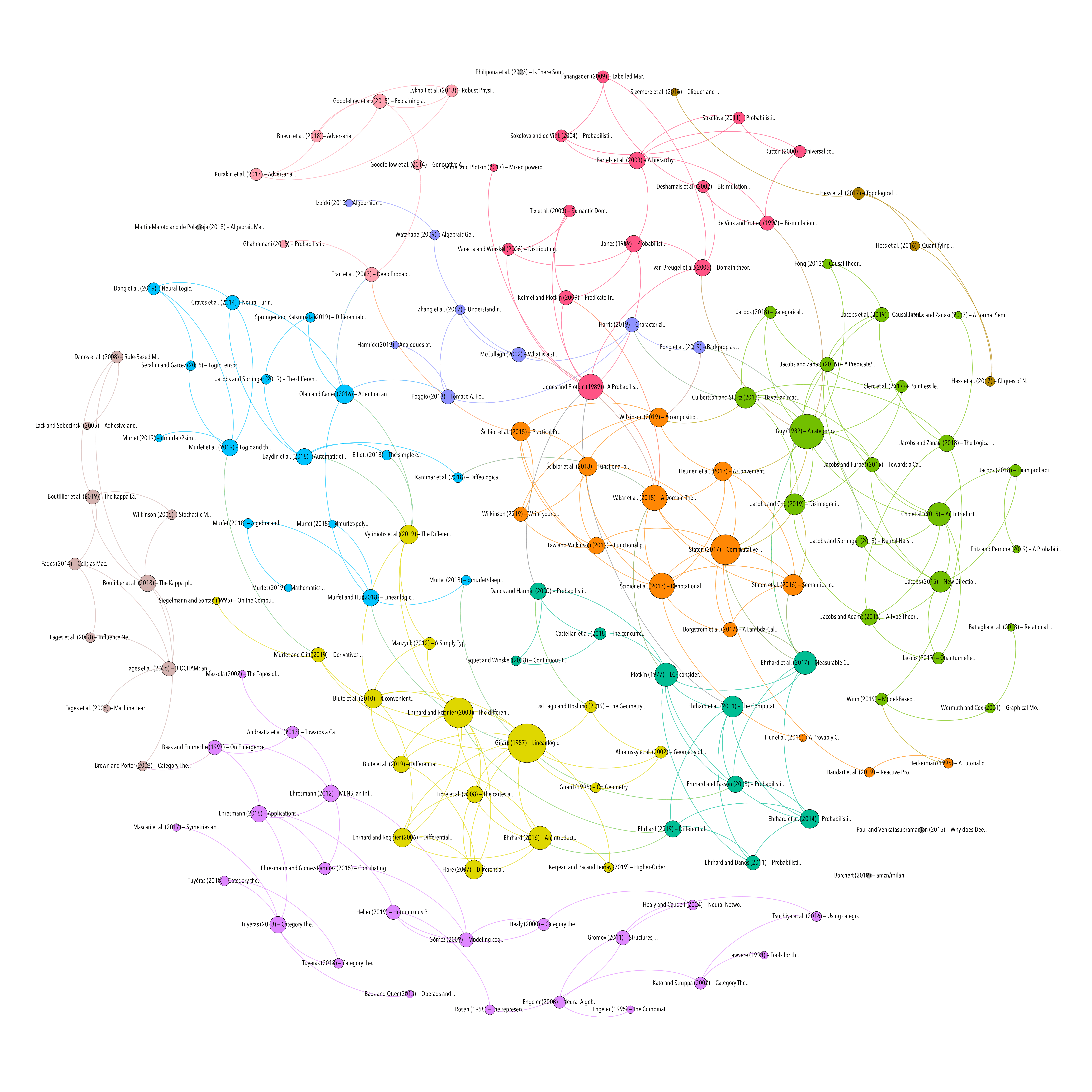Topic
Publication year

Resource type
Authors/contributors
Title
Abstract
We construct an operad $\mathrm{Phyl}$ whose operations are the edge-labelled trees used in phylogenetics. This operad is the coproduct of $\mathrm{Com}$, the operad for commutative semigroups, and $[0,\infty)$, the operad with unary operations corresponding to nonnegative real numbers, where composition is addition. We show that there is a homeomorphism between the space of $n$-ary operations of $\mathrm{Phyl}$ and $\mathcal{T}_n\times [0,\infty)^{n+1}$, where $\mathcal{T}_n$ is the space of metric $n$-trees introduced by Billera, Holmes and Vogtmann. Furthermore, we show that the Markov models used to reconstruct phylogenetic trees from genome data give coalgebras of $\mathrm{Phyl}$. These always extend to coalgebras of the larger operad $\mathrm{Com} + [0,\infty]$, since Markov processes on finite sets converge to an equilibrium as time approaches infinity. We show that for any operad $O$, its coproduct with $[0,\infty]$ contains the operad $W(O)$ constucted by Boardman and Vogt. To prove these results, we explicitly describe the coproduct of operads in terms of labelled trees.
Date
2015
Library Catalog
Semantic Scholar
Extra
ZSCC: NoCitationData[s1] arXiv: 1512.03337
Citation
Baez, J. C., & Otter, N. (2015). Operads and Phylogenetic Trees.
BIOLOGY, NEUROSCIENCE & PSYCHOLOGY
MODEL CHECKING AND STATE MACHINES
Topic
Processing time: 0.04 secondsGraph of references (from Zotero to Gephi via Zotnet with this script)